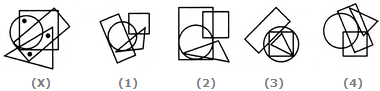# Non Verbal Reasoning - Dot Situation - Discussion

### Discussion :: Dot Situation - Section 1 (Q.No.2)

From amongst the figures marked (1), (2), (3) and (4), select the figure which satisfies the same conditions of placement of the dots as in figure (X).

2.

Select the figure which satisfies the same conditions of placement of the dots as in Figure-X.[A]. 1 [B]. 2 [C]. 3 [D]. 4

Explanation:

In fig. (X), one of the dots lies in the region common to the circle and the square only, another dot lies in the region common to the square, the triangle and the rectangle only and the third dot lies in the region common to the triangle and the rectangle only. In each of the figures (1), (2) and (3) there is no region common to the square, the triangle and the rectangle only. Only fig. (4) consists of all the three types of regions.